# Physical constantLight from the Sun traverses space at a constant speed before it reaches the Earth. The speed of light through a vacuum is an important physical constant and is thought to be the maximum speed any object or radiation can attain in the universe.

In physics, a physical constant is a physical quantity with a value that is generally believed to be both universal in nature and to remain unchanged over time. By contrast, a mathematical constant, which also has a fixed value, does not directly involve any physical measurement.

There are many physical constants in science. Some of the most widely recognized are:

• the rationalized Planck's constant ħ,
• the gravitational constant G,
• the speed of light in a vacuum c
• the electric constant ε0,
• the elementary charge e, and
• the fine-structure constant α.

Some fundamental physical constants (such as α above) do not have dimensions. Physicists recognize that if these constants were significantly different from their current values, the universe would be so radically different that stars like our Sun would not be able to exist and intelligent life would not have emerged.

## Physical constants with and without dimensional units

Many physical constants have units of dimension. For example, the speed of light in a vacuum, which is thought to be the maximum speed limit for any object or radiation in the universe, is expressed in the dimensions of distance divided by time. Its value is 299,792,458 meters per second (m·s-1). The universal gravitational constant (or Newton's constant) has been determined to be about 6.6742 × 10-11 cubic meters per kilogram per second squared (m3·kg-1·s-2).

Other physical constants are dimensionless. Among physicists, the best-known dimensionless physical constant is the fine structure constant α. Its value is currently measured as approximately 1/137.035999, but no one knows why it has this value. Many attempts have been made to derive this value theoretically, but so far none has succeeded. The same holds true for the dimensionless ratios of masses of fundamental particles, such as the ratio of the mass of the proton (mp) to that of the electron (me), which is approximately 1836.152673.

For physical constants that have units of dimension, their numerical values depend on the unit system used, such as SI or cgs (centimeters, grams, seconds). These units are set up by human convention. By contrast, the values of dimensionless constants are independent of any system of units, and in that sense they are independent of human convention. On that basis, theoretical physicists tend to regard these dimensionless quantities as fundamental physical constants. Yet, researchers may use the phrase "fundamental physical constant" in other ways as well. For example, the National Institute of Standards and Technology (NIST) uses it to refer to any universal physical quantity believed to be constant, such as the speed of light, c, and the gravitational constant, G.

While both mathematical constants and certain physical constants are dimensionless, those in the latter group are determined experimentally and are not defined by any combination of pure mathematical constants. Nonetheless, with the development of quantum chemistry in the twentieth century, a large number of previously inexplicable dimensionless physical constants have been successfully computed by theory. Also, the list of fundamental physical constants decreases when new theories show how some previously fundamental constant can be computed in terms of others. The list increases when experiments measure new effects.

### The fine structure constant, α

The fine structure constant, α, is dimensionless. It characterizes the strength of the electromagnetic interaction (electromagnetic force). The mathematical equation for this constant is: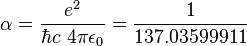$\alpha = \frac{e^2}{\hbar c \ 4 \pi \epsilon_0} = \frac{1}{137.03599911}$

where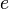$e \$ is the elementary charge,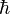$\hbar \$ is the reduced Planck's constant,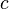$c \$ is the speed of light in a vacuum, and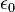$\epsilon_0 \$ is the permittivity of free space. In simple terms, the fine structure constant determines how strong the electromagnetic force is.

### Natural units

Physicists try to make their theories simpler and more elegant by reducing the number of physical constants appearing in the mathematical expression of their theories. This is accomplished by defining the units of measurement in such a way that several of the most common physical constants, such as the speed of light, are normalized to unity. The resulting system of units, known as "natural units," has a fair following in the literature on advanced physics because it considerably simplifies many equations.

## How many dimensionless physical constants are there?

A long-sought goal of theoretical physics is to reduce the number of fundamental (dimensionless) constants that need to be put in by hand, by calculating some from first principles. The reduction of chemistry to physics was an enormous step in this direction, because properties of atoms and molecules can now be calculated from the Standard Model, at least in principle. A successful "Grand Unified Theory" or "Theory of Everything" might reduce the number of fundamental constants further, ideally to zero. However, this goal remains elusive.

According to Michio Kaku (1994: 124-27), the Standard Model of particle physics contains 19 arbitrary dimensionless constants that describe the masses of the particles and the strengths of the various interactions. This was before it was discovered that neutrinos can have nonzero mass, and his list includes a quantity called the theta angle, which seems to be zero.

After the discovery of neutrino mass, and leaving out the theta angle, John Baez (2002) noted that the new Standard Model requires 25 arbitrary fundamental constants, namely:

• the fine structure constant,
• the strong coupling constant,
• the masses of the fundamental particles (normalized to the mass of some natural unit of mass), namely the masses of the 6 quarks, the 6 leptons, the Higgs boson, the W boson and the Z boson,
• the 4 parameters of the CKM matrix, which describe how quarks can oscillate between different forms,
• the 4 parameters of the Maki-Nakagawa-Sakata matrix, which does the same thing for neutrinos.

If we take gravity into account, we need at least one more fundamental constant, namely, the cosmological constant of Einstein's equations, which describe general relativity.

This gives a total of 26 fundamental physical constants. There are presumably more constants waiting to be discovered, describing the properties of dark matter. If dark energy turns out to be more complicated than a mere cosmological constant, even more constants will be needed.

In his book Just Six Numbers, Martin Rees considers the following numbers:

• Nu: ratio of the electroweak to the gravitational force;
• Epsilon: related to the strong force;
• Omega: the number of electrons and protons in the observable universe;
• Lambda: cosmological constant;
• Q: ratio of fundamental energies;
• Delta: number of spatial dimensions.

## How constant are the physical constants?

Beginning with Paul Dirac in 1937, some scientists have speculated that physical constants might decrease in proportion to the age of the universe. Scientific experiments have not yet provided any definite evidence for this, but they have indicated that such changes, if any, would be very small, and the experimental results have placed uppermost limits on some putative changes. For example, the maximum possible relative change has been estimated at roughly 10-5 per year for the fine structure constant α, and 10-11 for the gravitational constant G.

There is currently a debate   about whether changes in physical constants that have dimensions—such as G, c, ħ, or ε0—would be operationally meaningful. It is, however, generally agreed that a sufficient change in a dimensionless constant (such as α) would definitely be noticed. John D. Barrow (2002) gives the following explanation.

"[An] important lesson we learn from the way that pure numbers like α define the world is what it really means for worlds to be different. The pure number we call the fine structure constant and denote by α is a combination of the electron charge, e, the speed of light, c, and Planck's constant, ħ. At first we might be tempted to think that a world in which the speed of light was slower would be a different world. But this would be a mistake. If c, ħ, and e were all changed so that the values they have in metric (or any other) units were different when we looked them up in our tables of physical constants, but the value of α remained the same, this new world would be observationally indistinguishable from our world. The only thing that counts in the definition of worlds are the values of the dimensionless constants of Nature. If all masses were doubled in value you cannot tell because all the pure numbers defined by the ratios of any pair of masses are unchanged."

## Some philosophical ramifications

Some physicists have explored the notion that if the dimensionless fundamental physical constants differed sufficiently from their current values, the universe would have taken a very different form. For example, a change in the value of the fine-structure constant (α) by a few percent would be enough to eliminate stars like our Sun and to prevent the emergence of intelligent living organisms. It therefore appears that our universe is fine-tuned for intelligent life.

Those who endorse the "weak anthropic principle" argue that it is because these fundamental constants have their respective values, there was sufficient order and richness in elemental diversity for life to have formed, subsequently evolving the intelligence necessary to determine the values for these constants.

The values of the physical constants constrain any plausible fundamental physical theory, which must either be able to produce these values from basic mathematics or accept these constants a priori. Thus only some physical theories can be valid in our universe. Some physicists have therefore raised the theoretical possibility of the existence of multiple universes in which the fundamental physical constants have different values. There is, however, no experimental evidence for the existence of multiple parallel universes.

## Table of universal constants

Quantity Symbol Value Relative Standard Uncertainty
characteristic impedance of vacuum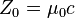$Z_0 = \mu_0 c \,$ 376.730 313 461... Ω defined
electric constant (permittivity of free space)$\epsilon_0 = 1 / ( \mu_0 c^2 )\,$ 8.854 187 817... × 10-12F·m-1 defined
magnetic constant (permeability of free space)$\mu_0 \,$ 4π × 10-7 N·A-2 = 1.2566 370 614... × 10-6 N·A-2 defined
Newtonian constant of gravitation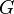$G \,$ 6.6742(10) × 10-11m3·kg-1·s-2 1.5 × 10-4
Planck's constant$h \,$ 6.626 0693(11) × 10-34 J·s 1.7 × 10-7
Dirac's constant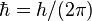$\hbar = h / (2 \pi)$ 1.054 571 68(18) × 10-34 J·s 1.7 × 10-7
speed of light in vacuum$c \,$ 299 792 458 m·s-1 defined

## Table of electromagnetic constants

Quantity Symbol Value1 (SI units) Relative Standard Uncertainty
Bohr magneton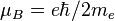$\mu_B = e \hbar / 2 m_e$ 927.400 949(80) × 10-26 J·T-1 8.6 × 10-8
conductance quantum$G_0 = 2 e^2 / h \,$ 7.748 091 733(26) × 10-5 S 3.3 × 10-9
Coulomb's constant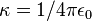$\kappa = 1 / 4\pi\epsilon_0 \,$ 8.987 742 438 × 109 N·m2C-2 defined
elementary charge$e \,$ 1.602 176 53(14) × 10-19 C 8.5 × 10-8
Josephson constant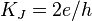$K_J = 2 e / h \,$ 483 597.879(41) × 109 Hz· V-1 8.5 × 10-8
magnetic flux quantum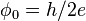$\phi_0 = h / 2 e \,$ 2.067 833 72(18) × 10-15 Wb 8.5 × 10-8
nuclear magneton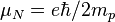$\mu_N = e \hbar / 2 m_p$ 5.050 783 43(43) × 10-27 J·T-1 8.6 × 10-8
resistance quantum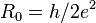$R_0 = h / 2 e^2 \,$ 12 906.403 725(43) Ω 3.3 × 10-9
von Klitzing constant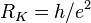$R_K = h / e^2 \,$ 25 812.807 449(86) Ω 3.3 × 10-9

## Table of atomic and nuclear constants

 Quantity Symbol Value1 (SI units) Relative Standard Uncertainty Bohr radius$a_0 = \alpha / 4 \pi R_\infin \,$ 0.529 177 2108(18) × 10-10 m 3.3 × 10-9 Fermi coupling constant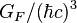$G_F / (\hbar c)^3$ 1.166 39(1) × 10-5 GeV-2 8.6 × 10-6 fine-structure constant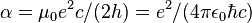$\alpha = \mu_0 e^2 c / (2 h) = e^2 / (4 \pi \epsilon_0 \hbar c) \,$ 7.297 352 568(24) × 10-3 3.3 × 10-9 Hartree energy$E_h = 2 R_\infin h c \,$ 4.359 744 17(75) × 10-18 J 1.7 × 10-7 quantum of circulation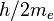$h / 2 m_e \,$ 3.636 947 550(24) × 10-4 m2 s-1 6.7 × 10-9 Rydberg constant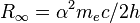$R_\infin = \alpha^2 m_e c / 2 h \,$ 10 973 731.568 525(73) m-1 6.6 × 10-12 Thomson cross section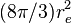$(8 \pi / 3)r_e^2$ 0.665 245 873(13) × 10-28 m2 2.0 × 10-8 weak mixing angle$\sin^2 \theta_W = 1 - (m_W / m_Z)^2 \,$ 0.222 15(76) 3.4 × 10-3

## Table of physicochemical constants

 Quantity Symbol Value1 (SI units) Relative Standard Uncertainty atomic mass unit (unified atomic mass unit)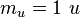$m_u = 1 \ u \,$ 1.660 538 86(28) × 10-27 kg 1.7 × 10-7 Avogadro's number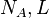$N_A, L \,$ 6.022 1415(10) × 1023 1.7 × 10-7 Boltzmann constant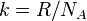$k = R / N_A \,$ 1.380 6505(24) × 10-23 J·K-1 1.8 × 10-6 Faraday constant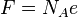$F = N_A e \,$ 96 485.3383(83)C·mol-1 8.6 × 10-8 first radiation constant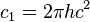$c_1 = 2 \pi h c^2 \,$ 3.741 771 38(64) × 10-16 W·m2 1.7 × 10-7 for spectral radiance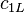$c_{1L} \,$ 1.191 042 82(20) × 10-16 W · m2 sr-1 1.7 × 10-7 Loschmidt constant at$T$=273.15 K and$p$=101.325 kPa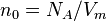$n_0 = N_A / V_m \,$ 2.686 7773(47) × 1025 m-3 1.8 × 10-6 gas constant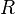$R \,$ 8.314 472(15) J·K-1·mol-1 1.7 × 10-6 molar Planck constant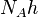$N_A h \,$ 3.990 312 716(27) × 10-10 J · s · mol-1 6.7 × 10-9 molar volume of an ideal gas at$T$=273.15 K and$p$=100 kPa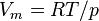$V_m = R T / p \,$ 22.710 981(40) × 10-3 m3 ·mol-1 1.7 × 10-6 at$T$=273.15 K and$p$=101.325 kPa 22.413 996(39) × 10-3 m3 ·mol-1 1.7 × 10-6 Sackur-Tetrode constant at$T$=1 K and$p$=100 kPa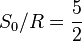$S_0 / R = \frac{5}{2}$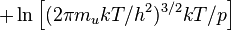$+ \ln\left[ (2\pi m_u k T / h^2)^{3/2} k T / p \right]$ -1.151 7047(44) 3.8 × 10-6 at$T$=1 K and$p$=101.325 kPa -1.164 8677(44) 3.8 × 10-6 second radiation constant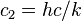$c_2 = h c / k \,$ 1.438 7752(25) × 10-2 m·K 1.7 × 10-6 Stefan-Boltzmann constant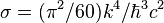$\sigma = (\pi^2 / 60) k^4 / \hbar^3 c^2$ 5.670 400(40) × 10-8 W·m-2·K-4 7.0 × 10-6 Wien displacement law constant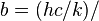$b = (h c / k) / \,$ 4.965 114 231... 2.897 7685(51) × 10-3 m · K 1.7 × 10-6

 Quantity Symbol Value (SI units) Relative Standard Uncertainty conventional value of Josephson constant2$K_{J-90} \,$ 483 597.9 × 109 Hz · V-1 defined conventional value of von Klitzing constant3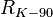$R_{K-90} \,$ 25 812.807 Ω defined molar mass constant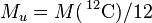$M_u = M(\,^{12}\mbox{C}) / 12$ 1 × 10-3 kg · mol-1 defined of carbon-12$M(\,^{12}\mbox{C}) = N_A m(\,^{12}\mbox{C})$ 12 × 10-3 kg · mol−1 defined standard acceleration of gravity (gee, free fall on Earth)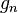$g_n \,\!$ 9.806 65 m·s-2 defined standard atmosphere$\mbox{atm} \,$ 101 325 Pa defined

## Notes for the above tables

1The values are given in the so-called concise form; the number in brackets is the standard uncertainty, which is the value multiplied by the relative standard uncertainty.
2This is the value adopted internationally for realizing representations of the volt using the Josephson effect.
3This is the value adopted internationally for realizing representations of the ohm using the quantum Hall effect.

## Footnotes

1. Note that Delta = 3. Being simply an integer, most physicists would not consider this a dimensionless physical constant of the usual sort.